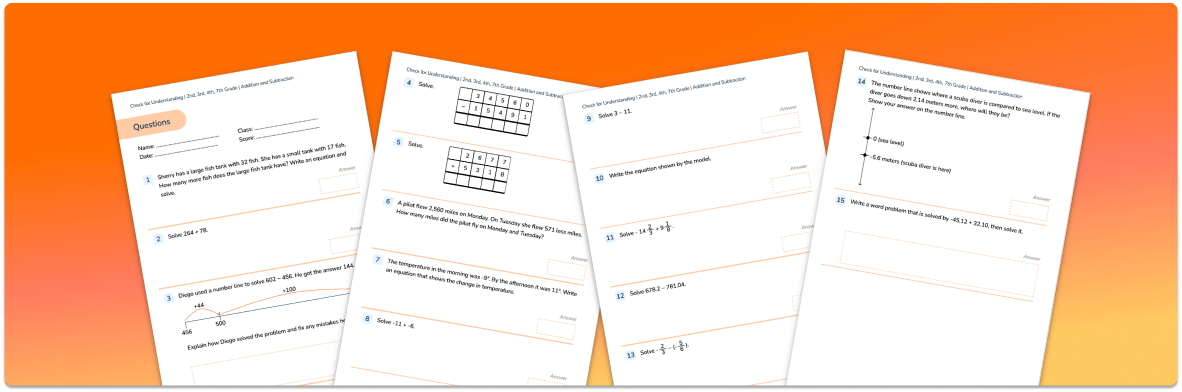Math Resources Quizzes

# Addition and Subtraction Check for UnderstandingUse this quiz to check your grade 2, 3, 4 and 7 students’ understanding of addition and subtraction.

• 15 questions with answers covering a range of 2nd, 3rd, 4th and 7th grade addition and subtraction topics.
• Identify areas of strength and where support is needed with topics such as the standard algorithm, subtracting with a number line and calculating with negative numbers.

You can unsubscribe at any time (each email we send will contain an easy way to unsubscribe). To find out more about how we use your data, see our privacy policy.

## Personalized one-on-one math instruction made easy

At Third Space Learning, we’re on a mission to help teachers like you shape your students into confident, able mathematicians.

Request a demo